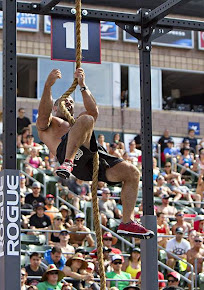## Pages2013 CROSSFIT GAMES!

### HERO WODS

ABATE =
ADAM BROWN =
ARNIE =
BADGER =
BLAKE =
BRENTON =
BULGER = 18:24
BULL = 38:37 – IN THE ICE
THE CHIEF WITH 185LB CLEAN AND RING PUSHUPS = 401 REPS
COE = 10:56
COLIN =
DANIEL = 13:47
DANNY = 5+50
DT = 9:51
ERIN =
FORREST =
GARRETT =
GRIFF=
HAMMER =
HANSEN =
HELTON =
HOLBROOK =
JACK = 13+10(1ST TIME) - 2ND TIME - 15+1
JASON =
JERRY =
JOHNSON =
JOSH =
JOSHIE =
JT = 6:12
KALSU = 21:22
LADESMA =
LUCE =
LUMBERJACK 20 = 21:24
MCCLUSKY = 25:08
MCGEE =
MICHAEL =
MOON =
MOORE =
MURPH =
NATE = 19+2
NUTTS = 14:24
PAUL = 19:28
RANDY =
RANKEL =
RJ =
RYAN = 11:15
ROY – 14:41
SEVENS =
SEVERINS =
SMALL =
STEPHEN =
THOMPSON =
TOMMY V = 12:16
TYLER =
WAR FRANK =
WEAVER =
WHITTEN = 32:19
WILMOT =
WITTMAN = 16:27
9/11 = 24:58